#### 期刊菜单

Simulation of Electric Vehicle Suspension Dynamic Characteristics
DOI: 10.12677/IaE.2019.72015, PDF, HTML, XML, 下载: 689  浏览: 2,176  国家科技经费支持

Abstract: Due to the change of the powertrain system, the suspension dynamic characteristics of the electric vehicle are different from those of the conventional car. In this paper, based on the relevant pa-rameters of an electric vehicle suspension system, a quarter-vehicle active suspension fuzzy con-troller is established in the Matlab/Simulink environment. The dynamic characteristics of the verti-cal acceleration of the vehicle body, the dynamic deflection of the suspension spring, and the dy-namic load of the tire are used as evaluation indexes for the effects of ride comfort and operational stability of vehicle. The simulation results show that the semi-active suspension with fuzzy control-ler has a good effect on the improvement of dynamic characteristics of electric vehicle suspension.

1. 引言

2. 四分之一悬架模型的建立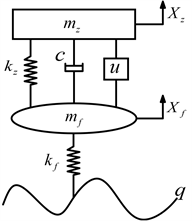Figure 1. 1/4 Car active suspension model

$\begin{array}{l}{m}_{z}{\stackrel{¨}{x}}_{z}+c\left({\stackrel{˙}{x}}_{z}-{\stackrel{˙}{x}}_{f}\right)+{k}_{z}\left({x}_{z}-{x}_{f}\right)-u=0\\ {m}_{f}{\stackrel{¨}{x}}_{f}-c\left({\stackrel{˙}{x}}_{z}-{\stackrel{˙}{x}}_{f}\right)-{k}_{z}\left({x}_{z}-{x}_{f}\right)+{k}_{f}\left({x}_{f}-q\right)+u=0\end{array}$ (1)

$\stackrel{˙}{q}\left(t\right)=-avq\left(t\right)+w\left(t\right)$ (2)3. 模糊控制器的设计

$\begin{array}{l}e=\left[-6,6\right]\\ {e}_{c}=\left[-6,6\right]\\ u=\left[-6,6\right]\end{array}$ (3)

$\begin{array}{l}{K}_{e}==40\\ {K}_{ec}=m/{e}_{c}=4\\ {K}_{u}=x/u=115\end{array}$ (4)Table 1. Fuzzy rule control table

4. 仿真分析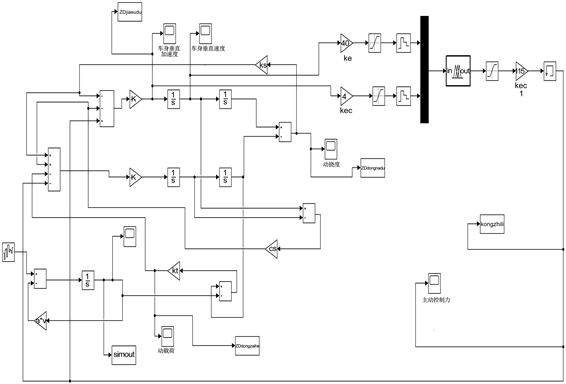Figure 3. Fuzzy control Simulink simulation model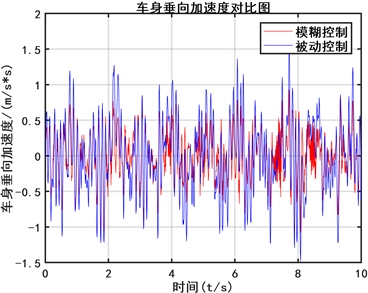Figure 4. Vertical acceleration of the car body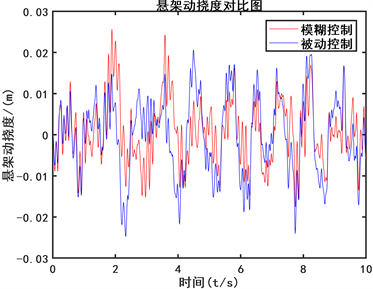Figure 5. Suspension deflection comparison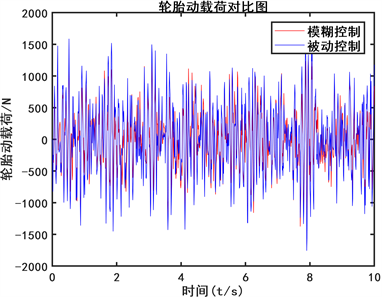Figure 6. Tire dynamic load comparisonTable 2. Comparison of smoothness evaluation indicators

5. 结论

1) 车辆行驶路面的不平度是随机性的，本文以随机白噪声作为系统的输入，来模拟随机路面，和实际路况相符。

2) 本文采用模糊控制的策略建立了四分之一车辆半主动悬架模型，通过本文提出的模糊控制策略控制主动控制力的实时输出，有效地改善了电动车悬架系统的的平顺性和操纵稳定性。验证了具有模糊控制器的半主动悬架对电动车悬架动态特性的改善有良好的效果。同时，模糊控制系统搭建简便，使得半主动悬架系统的建立具有实际意义。

NOTES

*通讯作者。

  喻凡, 郭孔辉. 车辆悬架的最优自适应与自校正控制[J]. 汽车工程, 1998, 20(4): 193-200.  喻凡, 郭孔辉. 自适应悬架对车辆性能改进的潜力[J]. 中国机械工程, 1998, 9(6): 67-69.  顾亮, 顾志强. 车辆悬架行驶平顺性全状态反馈主动控制[J]. 车辆与动力技术, 1998(2): 16-22.  丁科, 侯朝桢, 罗莉. 车辆主动悬架的神经网络模糊控制[J]. 汽车工程, 2001, 23(5): 340-343.  刘宏伟, 雷海蓉, 陈燕虹, 等. 空气悬架系统模糊控制仿真分析[J]. 汽车技术, 2003(7): 13.  刘静. 基于MATLAB/Simulink的车辆悬架建模与仿真[J]. 公路与汽运, 2011(6): 22-27.  Sun, Y.Q., Zhao, L.F. and Xiang, W. (2013) A Fuzzy Logic Controller for Vehicle-Active Suspension Systems. Journal of Hunan University, 805-806, 1645-1649. https://doi.org/10.4028/www.scientific.net/AMR.805-806.1645  Lian, R.J., et al. (2005) Self-Organizing Fuzzy Control of Active Suspension Systems. International Journal of Systems Science, 36, 119-135. https://doi.org/10.1080/00207720512331338102  陈杰平, 冯武堂, 郭万山, 等. 整车磁流变减振器半主动悬架变论域模糊控制策略[J]. 农业机械学报, 2011, 42(5): 7-13.  檀润华, 陈鹰, 路甬祥. 路面对汽车激励的时域模型建立及计算机仿真[J]. 中国公路学报, 1998, 11(3): 96-102.